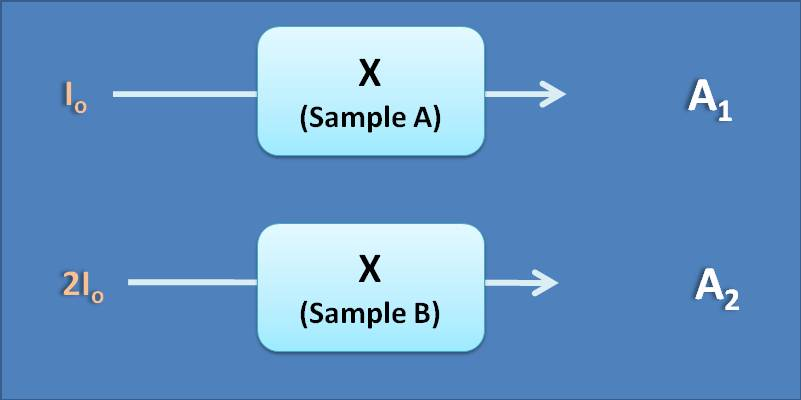1. Home
2. â€º
3. Test papers
4. â€º

# MCQ on UV-Visible spectroscopy: Page-9

Molar extinction coefficient has units of

(A) lit.mol-1.cm-1

(B) dl.g -1cm -1

(C) No units

(D) lit.g -1cm -1

2. Two samples each containing same analyte at equal concentration are irradiated with different intensities of radiation as shown below. Select the CORRECT statement regarding the absorption shown by two samples.(A) A 2=4 A 1

(B) A 2=2 A 1

(C) A 2=A 1

(D) A 2=1/2 A 1

Absorbance is independent of intensity of the incident radiation as it is the ratio between intensities of incident radiation (I o) and transmitted radiations (I t). If you double I o, then I t will also be doubled proportionally making absorbance remains constant.

3. Which of the following statement is not correct ?

(A) Absorptivity changes with the intensity of the light

(B) Absorbance is independent of intensity of the light

(C) Absorbance has no units

(D) Absorptivity is a constant and depends on the nature of the molecule

Absorptivity is the intrinsic property of a molecule and it will be a constant at a specific temperature and wavelength of radiation. So it doesn’t change with intensity of incident radiation at a particular wavelength. that

4. The possible transitions in the following compounds are(A) n→π*, π→π*

(B) σ→σ*, n→π*, π→π*

(C) σ→σ*, n→σ*

(D) n→π*, n→σ*

All the listed compounds contain similar type of electrons such as sigma and n electrons. Therefore they can produce two types of transitions such as s→s* and n→s*. Both these transitions fall below 200 nm therefore show absorption only in vacuum UV region. These compounds can’t show p→p* and n→p* as they don’t have pi electrons.

5. The above listed compounds are transparent in

(A) Vacuum UV region

(B) UV-visible region

(C) Visible only

(D) UV only

Since they doesn’t absorb in UV-visible region, they are transparent and colourless in this region. For a compound to absorb in UV-Visible region, it should have pi electrons.